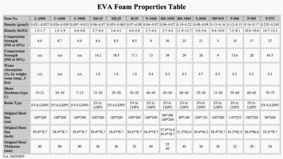### TENSILE STRENGTH

The tensile strength of a material is the maximum amount of tensile stress that can be applied to it before it ceases to be elastic.

Tensile strength is measured in units of force per unit area. The unit is newton per square meter (N/m^2), kilogram (force) per square centimeter (kg/cm^2) or pounds per square inch (psi).

The ultimate tensile strength (UTS) of a material is the force per unit area at which it breaks in two.More technical data of EVA foam material